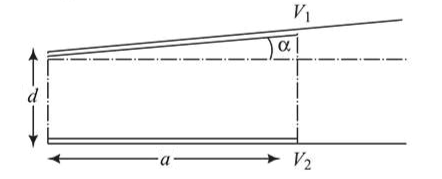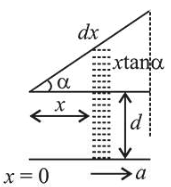# A capacitor is made of two square plates each of side 'a ' making a very small angle a between them,Question:

A capacitor is made of two square plates each of side ' $a$ ' making a very small angle a between them, as shown in figure. The capacitance will be close to:1. (1) $\frac{\in_{0} a^{2}}{d}\left(1-\frac{\alpha a}{2 d}\right)$

2. (2) $\frac{\in_{0} a^{2}}{d}\left(1-\frac{\alpha a}{4 d}\right)$

3. (3) $\frac{\epsilon_{0} a^{2}}{d}\left(1+\frac{\alpha a}{d}\right)$

4. (4) $\frac{\in_{0} a^{2}}{d}\left(1-\frac{3 \alpha a}{2 d}\right)$

Correct Option: 1,

Solution:Consider an infinitesimal strip of capacitor of thickness $\mathrm{d} \mathrm{x}$ at a distance $\mathrm{x}$ as shown.

Capacitance of parallel plate capacitor of area $\mathrm{A}$ is given by $C=\frac{\varepsilon_{0} A}{t}$

[Here $t=$ seperation between plates $]$

So, capacitance of thickness $\mathrm{dx}$ will be

$\therefore d C=\frac{\varepsilon_{0} a d x}{d+x \tan \alpha}$

Total capacitance of system can be obtained by integrating with limits $\mathrm{x}=0$ to $\mathrm{x}=\mathrm{a}$

$\therefore C_{e q}=\int d C=a \varepsilon_{0} \int_{x=0}^{x=a} \frac{d x}{x \tan \alpha+d}$

[By Binomial expansion]

$\Rightarrow C_{e q}=\frac{a \varepsilon_{0}}{d} \int_{0}^{a}\left(1-\frac{x \tan \alpha}{d}\right) d x=\frac{a \varepsilon_{0}}{d}\left(x-\frac{x^{2} \tan \alpha}{2 d}\right)_{0}^{a}$

$\Rightarrow C_{e q}=\frac{a^{2} \varepsilon_{0}}{d}=\left(1-\frac{a \tan \alpha}{2 d}\right)=\frac{\varepsilon_{0} a^{2}}{d}\left(1-\frac{\alpha a}{2 d}\right)$